# Area of a Plot of Land

The last four posts dealt with formulas for finding areas using lengths of sides, starting with the triangle, where that is all you need, and then quadrilaterals, where something more must be added; and then using coordinates of vertices. Now we can use those tools to solve some of the more common real-life problems we have received: finding the area of a plot of land. There will be several methods, depending on what data you have in addition to lengths.

## Four sides and a diagonal

Here is a question from 2002:

Area of a Lawn

I have two areas of lawn that I want to sod with new grass, and I don't know how much sod to buy. One lawn is 14 yds wide at one end and 7 yds wide at the other end. The lengths are 16 yds and 20 yds. The other lawn is 30 yds long by 32 yds long by 5 yds wide by 10 yds wide.

The sod comes in squares. I can easily cut the sod with a shovel. Let me try to better describe the lawn(s). If you draw on paper the following. Top of the lawn is 14 yds across. The bottom side is 7 yds across. The left side runs 16 yds and the right side runs 22 yds. On paper the diagonal from the top right corner down to the bottom left corner is approx 20 yds. From the top left corner down to the bottom right corner is approx 22 yds. On the other lawn, the top of the lawn is 10 yds across. The bottom side is 5 yds across. The left side runs 30 yds and the right side runs 32 yds. The diagonal from the top right corner down to the bottom left is approx 31 yds. From the top left corner down to the bottom right is approx 33 yds.

The initial description, giving only the “lengths” and “widths” (as if it were a rectangle) was insufficient; but Randy wisely gave us just what we have asked others for: the lengths of diagonals, too. That makes it possible to make a scale drawing; here is what the first looks like: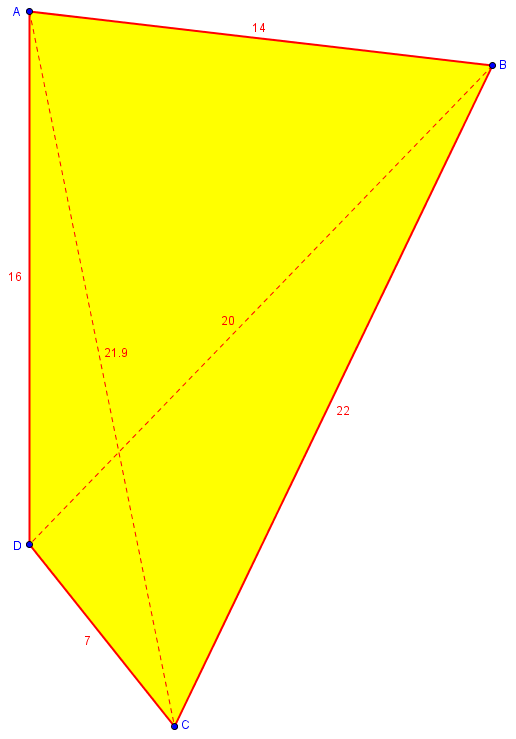Note that if diagonal BD is exactly 20 yards, AC is not exactly 22. Both were described as approximate, so this is the best we can do. The figure is “overspecified”, with a slight conflict among the numbers; but the fact that it comes out so close helps give confidence that the numbers are all reasonably accurate. We will find such confirmation useful in many problems of this type.

Doctor Jeremiah answered, first introducing Heron’s formula that we have seen before:

Let's calculate the area, and after that we can calculate the number of squares.

The area of a triangle can be found like this:

Assume that the lawn can be cut along a diagonal to make a triangle.  Then we can use Heron's formula to find the area

+-----------------a-----------------+
\                               +
\                           +
\                       +
\                   +
b               c
\           +
\       +
\   +
+

First calculate the intermediate value: s = (a+b+c)/2 Then the area is:  Area = sqrt[s(s-a)(s-b)(s-c)] where sqrt[x] is the square root of x.

Since either diagonal divides the polygon into two triangles, we just have to apply this twice. First, my ABD:

So for your first lawn we will work on the top left triangle and then the lower right triangle.

The top of the lawn is 14 yds (call that value 'a').
The left side runs 16 yds (call this value 'b').
The third side of the triangle is the diagonal.
The diagonal from the top right corner down to the    bottom left corner is approx 20 yds (call this 'c').

Then s = (a+b+c)/2 = (14+16+20)/2 = 50/2 = 25 square yards, and thearea of this triangle is sqrt[s(s-a)(s-b)(s-c)]. If we plug in a, b, c, and s we get:
sqrt[s(s-a)(s-b)(s-c)]
sqrt[25(25-14)(25-16)(25-20)]
sqrt[25(11)(9)(5)]
sqrt
upper-left triangle area: 111.24 square yards

Next, my BCD:

Now we do the other triangle that makes up the lawn:

The bottom side is 7 yds across (call that 'a').
The right side runs 22 yds (call this 'b').
The diagonal from the top right corner down to the  bottom left corner is approx 20 yds (this is 'c').

Then s = (a+b+c)/2 = (7+22+20)/2 = 49/2 = 24.5 square yards, and the area of this triangle is sqrt[s(s-a)(s-b)(s-c)]. If we plug in a, b, c, and s we get:
sqrt[s(s-a)(s-b)(s-c)]
sqrt[24.5(24.5-7)(24.5-22)(24.5-20)]
sqrt[24.5(17.5)(2.5)(4.5)]
sqrt[4823.4375]
lower-right triangle area: 69.45 square yards

This means the total area of the lawn is the sum of the two triangular areas, a total of 180.69 square yards. If 1 square of sod is 1 square yard, then you need 181 squares of sod (because you can't buy 0.69 squares of sod).

We could also use the other diagonal. Here is my work for that, using the 22 yards that Randy stated, which means the area will probably be a little different:

$$K_{ABC} = \sqrt{(29)(15)(7)(7)} = \sqrt{21315} = 146.00;$$

$$K_{ACD} = \sqrt{(22.5)(6.5)(15.5)(0.5)} = \sqrt{1133.44} = 33.67;$$

$$K_{ABCD} = K_{ABC} + K_{ACD} = 179.67.$$

This differs from the other calculation by only 1 square yard, which is good.

The other lawn would be done the same way. And in general the area of anything can be calculated if you can break it up into triangles.

Here is a picture: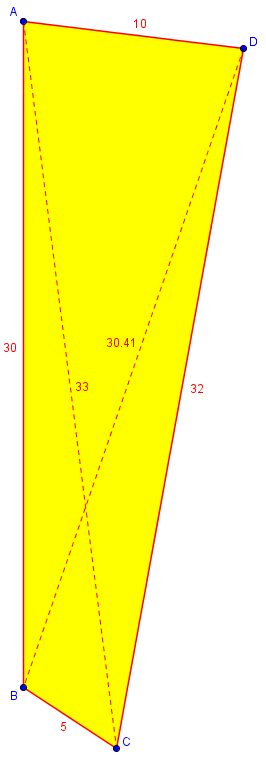Again, the second diagonal, BD, doesn’t match up quite right, but it’s good enough. I’ll leave this one for you to do. (GeoGebra gives the area as 222.56, if you want to check.)

A similar method (with extra ideas for calculating the angles) is found here:

Carpeting an Irregular Hexagon

## Four sides and one (right) angle

Consider this question from 2003:

Determining Area of a Lot of LandI own a lot that we are trying to figure out the square footage for. There are various records that show various results, but the following is the best record I have of the dimensions. Could you please provide the answer and the formula? The dimensions are 98.20' x 100.89' x 140.79' x .89'

Gary gave only the lengths of the sides, which as we know is insufficient. But in drawing this one just now, I realized that one side is far shorter than the others, making this practically a triangle. If we didn’t want precision, we could just apply Heron’s formula to the sides 98.20′, 100.89′, and 140.79′: We have $$s = 169.94$$, so $$K = \sqrt{(169.94)(71.74)(69.05)(29.15)} = \sqrt{24539133.78} = 4953.7.$$

Doctor Rob took the question:

You have not given enough information to solve the problem. The best that I could tell you is that the area is *at most* 5016.35 square feet. In order to answer your question exactly, you will have to give at least one of the angles, or a diagonal distance, or some additional data. For example, are two of the sides parallel? Is the area convex?...It turns out the maximum area occurs when the four corners of the lot lie on the circumference of a single circle. That's how I could compute the maximum area in your problem.

He found the maximum possible area using Brahmagupta’s formula for the cyclic quadrilateral: $$s = (98.20+100.89+140.79+0.89)\div 2 = 170.385\\ K = \sqrt{(s-a)(s-b)(s-c)(s-d)}\\ = \sqrt{(72.185)(69.495)(29.595)(169.495)}\\ = \sqrt{25163772.82} = 5016.35.$$

Gary replied with an angle:

I appreciate your taking the time to respond. I do have one 90-degree angle at the intersection of the sides that measure 98.20' x 100.89'.

That one angle is enough (and, in fact, any configuration of these sides will come very close to a right angle there).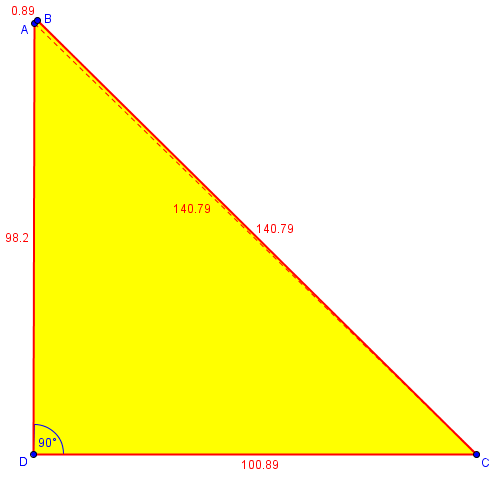Doctor Rob could now answer the question, by finding the area of the right triangle, then using its hypotenuse in Heron’s formula for the other (very thin) triangle:

Thanks for writing back to Ask Dr. Math, Gary.

Okay! The right triangle formed at that corner by drawing the other diagonal has hypotenuse of length

L = sqrt(98.20^2 + 100.89^2) = 140.79073... feet.

Then the area of the right triangle is

A1 = (1/2)*b*h,
= 0.5*100.89*98.20,
= 4953.699 square feet,

and the area of the other triangle, using Heron's Formula, is

A2 = sqrt[s*(s-a)*(s-b)*(s-c)],

where

a = 140.79073 feet,
b = 140.79 feet,
c = 0.89 feet,
s = (a+b+c)/2 = 141.235365 feet,

so

A2 = sqrt(141.235365*0.444635*0.445365*140.345365),
= sqrt(3925.195215...),
= 62.651378... square feet.

Then the total is

A = A1 + A2 = 5016.350378... square feet,

which equals the maximum possible value 5016.35054787... to three decimal places (but not exactly).

Feel free to write again if I can help further.

If the one known angle had been something other than 90 degrees, we could use trigonometry to find the first triangle’s area and sides, so this illustrates a more general method.

A purely trigonometric method using angles is described here by Doctor Ken:

Area of an Irregular Polygon

## Two angles to each vertex

Surveyors, as we know from observing them at work, focus on finding angles, rather than distances. The next question, from 1999, is about how that works:

Area of PolygonI found it stated in a popular little paperback on math that a surveyor can calculate the area of a polygon this way:

1. Lay out and measure a line of any length   within the polygon (the "base line").

2. Measure the angle to each vertex of the   polygon from each end of the line.

The resulting length of the single line and the angles supposedly contain sufficient information to compute the area.

In trying to solve this I've been able to compute the areas of triangles that have as one of their sides the base line, and I've been able to solve some, but not all, of the other triangles. I'm stuck on a few peripheral triangles for which I can't seem to get enough information to solve.

Is there a general algorithm to solve this problem for polygons of any number of sides; convex, concave, or when you do not have the lengths of any sides? If I can ever figure this out, I want to write a program to do the calculations.

There are several ways to do this; taking a clue from the fact that the Shoelace Formula I discussed last time is also called the Surveyor’s Formula, I suggested a way to use trigonometry to find coordinates to put into that formula:

It's obvious that there is enough information in the angles and the baseline, because they are sufficient to draw the figure (using ASA repeatedly), and therefore the area is determined by these numbers. What you want to know, of course, is HOW?

I'm not familiar with any traditional method used by surveyors, but I can at least come up with a method that will work. It takes two steps.

First, if we choose a coordinate system with one end of the baseline as the origin and the other as point (d,0), we can find the coordinates of each vertex. I used this figure:

P
+
/| \
/ |   \
/  |     \
/   |y      \
/    |         \
/     |           \
/a     |            b\
+-------+---------------+
A   x        d-x        B

to write two equations

y/x = tan(a)
y/(d-x) = tan(b)

from which I got the formulas

d                        d
x = ----------   and   y = ---------------
tan(a)                   1        1
------ + 1             ------ + ------
tan(b)                 tan(a)   tan(b)

You can check those; I did it pretty quickly, but it gives the idea. The cases where the tangents are 0 or infinite can be handled easily.The second step is to use these coordinates for each point (x_n,y_n) to find the area. There is a standard formula for this:

A = [(x1y2 + x2y3 + ... + xny1) - (y1x2 + y2x3 + ... + ynx1)]/2

I think that should be enough - maybe not as elegant as it could be, but pretty straightforward.

Since there are no specific numbers there, let’s make an example. I’ve drawn a pentagon and measured the angles from each end of side AB to the other vertices, C, D, and E: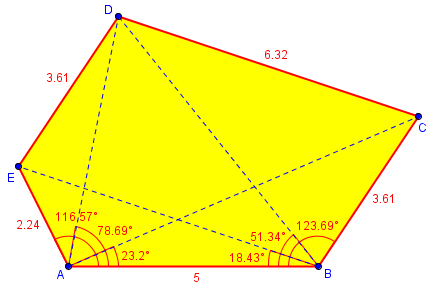Our base line is 5 meters; we can take point A as (0, 0), and B as (5, 0). For point C, the angles are 23.2° and 123.69°, so we find $$x = \frac{d}{\frac{\tan \alpha}{\tan \beta} + 1} = \frac{5}{\frac{\tan 23.2°}{\tan 123.69°} + 1} = \frac{5}{\frac{0.4286}{-1.5000} + 1} = 7.000$$ $$y = \frac{d}{\frac{1}{\tan \alpha}+\frac{1}{\tan \beta}} = \frac{5}{\frac{1}{\tan 23.2°}+\frac{1}{123.69°}} = \frac{5}{\frac{1}{0.4286}+\frac{1}{-1.5000}} = 3.000.$$ (Yes, I chose my points with simple coordinates, unlike real life.) So C is (7, 3). Similarly, D is (1, 5) and E is (-1, 2). Using the Shoelace Formula, we put the five ordered pairs into an array (with the first copied at the bottom), $$\begin{bmatrix}0 & 0\\ 5 & 0\\ 7 & 3\\ 1 & 5\\ -1 & 2\\ 0 & 0\end{bmatrix}$$

Then our area is $$K = \frac{1}{2}[(0+15+35+2+0) – (0+0+3-5+0)] = 27 \text{ square meters.}$$

## Survey data: lengths and directions

We’ll look at one more type of input: the actual data surveyors put on what is called a plat map.

Land Plot AreaI have a parcel of land that is a trapezoid. The four sides are not equal.

The road frontage is 363.04 ft in a northeasterly direction.

One side is 1950.16 ft in a southeasterly direction.

The back is 338.49 ft in a southwesterly direction.

The final side is 2000.75 ft in a northwesterly direction.

The surveyor has determined it to be 624,630.88 square ft and 14.340 acres overall. The legal description is 15.8 acres.

How can I figure out the square footage?

Here we have four lengths, and general directions — close to what we need.

I replied:

You call this a trapezoid, but I suspect you do not mean that two sides are exactly parallel, or you would probably have said so. Without knowing at least one exact angle (better than "northeast"), I can't find the exact area. Do you know the bearing along each side?

The square feet you give do convert to 14.340 acres; and 15.8 acres is very close to what you get by just multiplying the average "length" by the average "width," which may suggest that the area was originally found by a simplistic and inaccurate method, or it may be correct if the angles are just right.

Please send me some angle data, and I can give you a better answer.

Tom responded (as few have ever done) with all the data:

The angles and measurements that are on the plat are as follows:

363.04 ft -- N 38 deg 15' 00" E
1950.16 ft -- plat reads: S 80 deg 36' 34" E
338.47 ft -- plat reads: S 30 deg 59' 49" W
200.75 fr -- N 80 deg 42' 11" W

Hope this helps; that's all the plat has on it.

For each side, we have not only the length, but the direction. The notation, if you are unfamiliar with it, means this:

• N 38° 15′ 00″ E means start pointing North, and turn toward East by 38 degrees, 15 minutes, and 00 seconds

Since this was such a good example, I wrote up my work in detail for future use (and also made a spreadsheet that has seen a lot of use for this sort of question, and has been improved over the years).

Okay, let's first convert the angles from surveyors' terms to mine. I'll measure angles clockwise from north, and put them in decimal form (the form "N x E" means we start at north and go x degrees toward the east):

AB: N 38 deg 15' 00" E --> 38.25 deg
BC: S 80 deg 36' 34" E --> 180-80.61 = 99.391 deg
CD: S 30 deg 59' 49" W --> 180+30.997 = 210.997 deg
DA: N 80 deg 42' 11" W --> 360-80.703 = 279.297 deg

This should look something like

B----_____ 1950
363/          -----_____
/                     ----C
A----_____                /
-----_____     /338
2000      ----D

Here is a version of the figure on GeoGebra: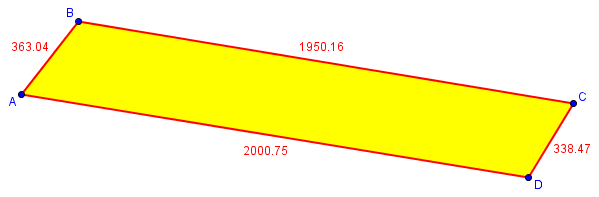Now we can turn each side into a vector, indicating how far N and E it goes; the north component is L cos(A) where L is the length of the side and A is the angle from north, and the east component is L sin(A).

Side   Length   Angle     North     East
----   ------   -----     -----     ----
AB    363.04   38.250   285.101   224.756
BC   1950.16   99.391  -318.195  1924.026
CD    338.47  210.997  -290.135  -174.309
DA   2000.75  279.297   323.224 -1974.469

For instance, to get from A to B, we go 285 feet north and 224 feet east.

Now we call A (0, 0), and add up to find the coordinates of each point (which I wrote, for some reason, as north, y, followed by east, x).

From here we can find the coordinates of each vertex relative to A:

North     East
-----     ----
A     0         0
B   285.101   224.756
C   -33.094  2148.782
D  -323.229  1974.473
(A)    -.005      .004

I included the location we get back to when we come to A, to check the accuracy of the calculations, and it looks pretty good.

If the final coordinates were not close to zero, we would know some measurement was wrong, because the figure would not close. This is a benefit of having more information than the 5 or 6 facts we need for a quadrilateral.

Finally, having coordinates of each vertex, I can use the Surveyor’s Formula (Shoelace Formula):

Now the last step is to find the area using this formula from our FAQ on analytic geometry:

http://mathforum.org/dr.math/faq/formulas/faq.ag2.html#twopolygons

K = [(x1y2 + x2y3 + x3y4 + ... + xny1) -
(x2y1 + x3y2 + x4y3 + ... + x1yn)]/2

This makes the area

K = [(0*224.756 + 285.101*2148.782 + -33.094*1974.473 + -323.229*0)
- (285.101*0 + -33.094*224.756 + -323.229*2148.782 + 0*1974.473)]
/2

= [547276.687 - -701986.732]/2 = 1249263.419/2
= 624631.709 ft^2

This, divided by 43560 ft^2 per acre, gives 14.34 acre.

Not surprisingly, the surveyor appears to have used his own data correctly.

A similar method is described here by Doctor Jerry:

Calculating Polygon Area

This site uses Akismet to reduce spam. Learn how your comment data is processed.